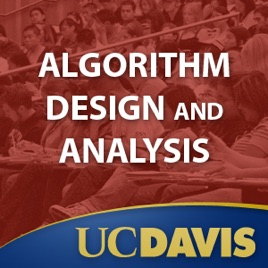30 episodes

The purpose of this undergraduate course is to introduce fundamental techniques and viewpoints for the design and the analysis of efficient computer algorithms, and to study important specific algorithms. The course relies heavily on mathematics and mathematical thinking in two ways: first as a way of proving properties about particular algorithms such as termination, and correctness; and second, as a way of establishing bounds on the worst case (or average case) use of some resource, usually time, by a specific algorithm. The course covers some randomized algorithms as well as deterministic algorithms.# Algorithm Design and Analysis UC Davis

• Technology

The purpose of this undergraduate course is to introduce fundamental techniques and viewpoints for the design and the analysis of efficient computer algorithms, and to study important specific algorithms. The course relies heavily on mathematics and mathematical thinking in two ways: first as a way of proving properties about particular algorithms such as termination, and correctness; and second, as a way of establishing bounds on the worst case (or average case) use of some resource, usually time, by a specific algorithm. The course covers some randomized algorithms as well as deterministic algorithms.

• video
Introduction to the videos

## Introduction to the videos

This video shows the URL for printed material that accompanies
the course, and a URL for more advanced lectures on
material that overlaps and extends the course.

The URL for printed course material is:
www.cs.ucdavis.edu/~gusfield/itunesU

The URL for more advanced lectures is:
www.cs.ucdavis.edu/~gusfield/cs222f07/videolist.html

• 2 min
• video
Introduction to the course and algorithm complexity

## Introduction to the course and algorithm complexity

This is the course introduction about algorithm complexity, including what "worst case running time" means and how it is measured.

• 49 min
• video
Big-Oh, Omega and Theta notation

## Big-Oh, Omega and Theta notation

In Lecture 2, Gusfield discusses Big-Oh, Omega and Theta notation. He describes Mergesort and Merge and the start of their time analysis.

• 48 min
• video
Time analysis of Mergesort

## Time analysis of Mergesort

In Lecture 3, Gusfield gives the worst-case analysis of MergeSort by setting up a recurrence relation and solving it by unwrapping.

• 49 min
• video
A more complex recurrence relation and counting inversions

## A more complex recurrence relation and counting inversions

In Lecture 4, students learn about solving a more complex recurrence relation by unwrapping. Gusfield also addresses the problem of counting inversions in a permutation.

• 52 min
• video
Counting inversions; Fast integer multiplication

## Counting inversions; Fast integer multiplication

Lecture 5: Gusfield lectures about counting the number of inversions in a permutation. He introduces fast integer multiplication by divide and conquer.

• 48 min

4.1 out of 5
10 Ratings

10 Ratings